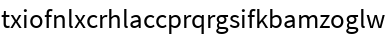#Function Repository Resource:

# VigenereCipher

Perform Vigenère's substitution cipher on a string

Contributed by: Sander Huisman
 ResourceFunction["VigenereCipher"][string,key] enciphers string using the key key. ResourceFunction["VigenereCipher"][string,{key1,key2,…}] repeatedly enciphers string using first key1, then key2, etc. ResourceFunction["VigenereCipher"][{s1,s2,…},key] enciphers the strings s1,s2, etc. using the key key. ResourceFunction["VigenereCipher"][key] represents an operator form of ResourceFunction["VigenereCipher"] that can be applied to an expression.

## Details and Options

The key can be in either lowercase or uppercase letters.
Spaces in the key will leave letters unchanged, as do the letters A and a in the key.
Letters outside az and AZ are not transformed.
The case of the original message is retained in the enciphered message.
When multiple keys are given and the LCM of the lengths of the keys is small, the keys get combined into a single key, which is then used to encipher string. This will, especially for long strings, yield faster results and give the same result as Fold[ResourceFunction["VigenereCipher"],string,{key1,key2,}].
ResourceFunction["VigenereCipher"][key][string] is equivalent to ResourceFunction["VigenereCipher"][string,key].

## Examples

### Basic Examples

Encipher a message using the key "LEMON":

 In:=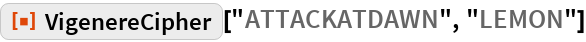Out=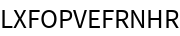Encipher with multiple keys:

 In:=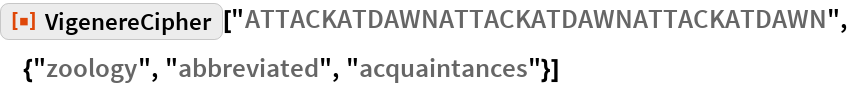Out=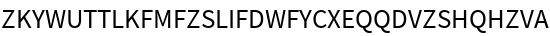### Scope

Encipher a message twice, first using "GO" and then "CAT":

 In:=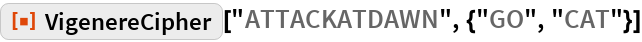Out=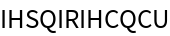Encipher multiple strings:

 In:=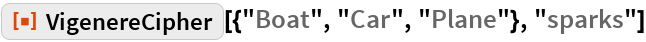Out=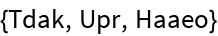Create an operator that enciphers strings with the key "sparks":

 In:=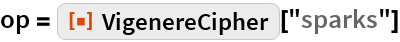Out=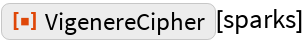Apply the operator to a string:

 In:=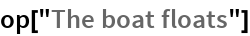Out=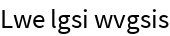### Properties and Relations

If a single character is used, the Vigenère cipher is identical to the Caesar cipher:

 In:=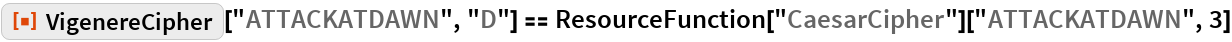Out=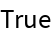When enciphering with multiple keys, the keys can first be enciphered with each other to encipher the message with a single key. The length of the replacing key has to be the LCM of the lengths of each of the keys:

 In:=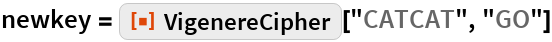Out=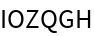This indeed matches with what you expect:

 In:=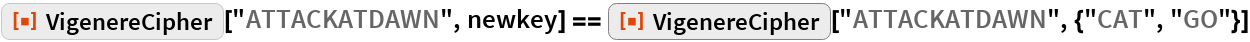Out=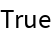The case of the original text is retained; the case of the key does not matter:

 In:=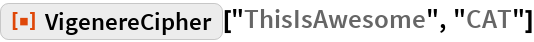Out=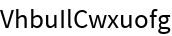In:=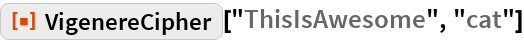Out=### Possible Issues

Spaces and the letters a and A in the key will not alter the original message:

 In:=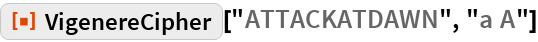Out=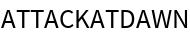The key should have valid letters and valid length:

 In:=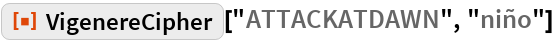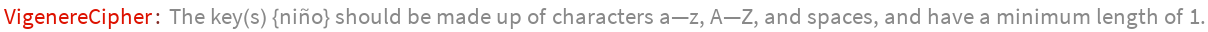Out=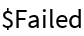In:=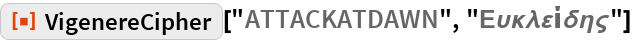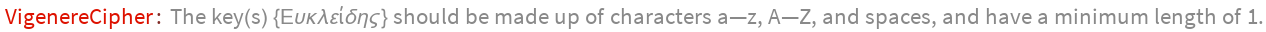Out=In:=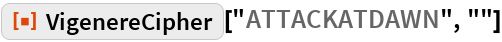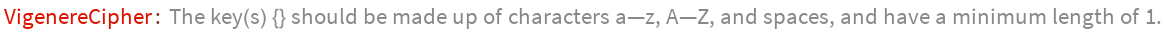Out=Two keys can negate each other:

 In:=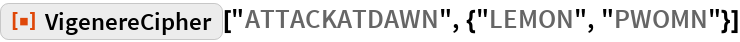Out=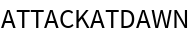Enciphering the keys with each other gives a string with only As:

 In:=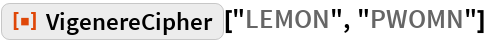Out=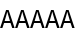Spaces, numbers and letters outside the regular English alphabet are not transformed:

 In:=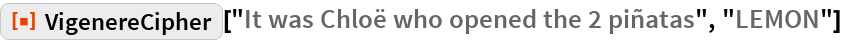Out=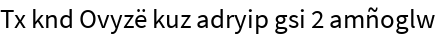Use RemoveDiacritics, StringDelete, IntegerName and ToLowerCase to transform the message:

 In:=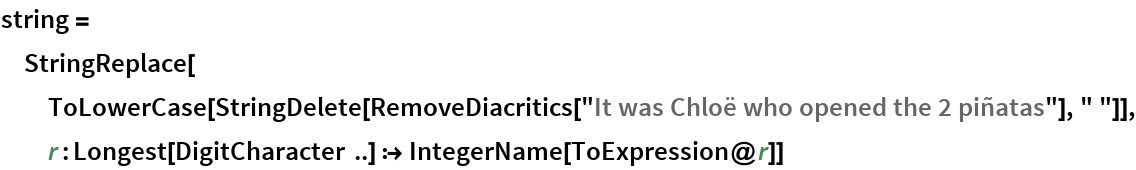Out=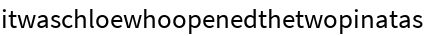Enciphering the transformed message results in a much-harder-to-crack cipher:

 In:=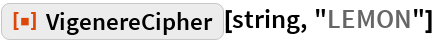Out=